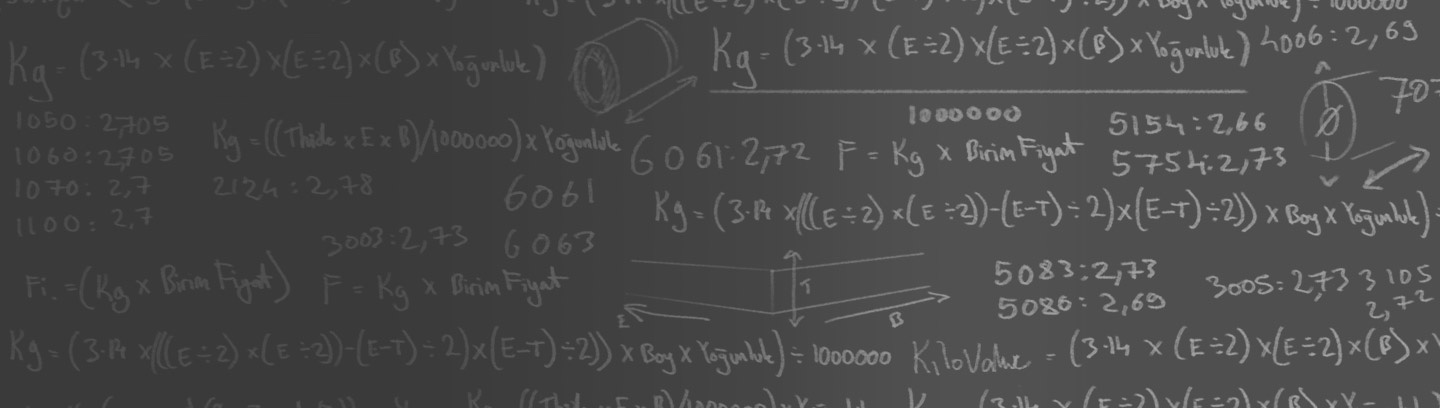# Aluminium Weight Calculator

### With our aluminium weight calculator, you can quickly calcuate the theoretical weight of our products.Don’t lose any more time with the aluminium weight formula!
1. Choose the Material
Cast Plate
Milled Plate
Rolled Plate
Sheet
Shate
Shipbuilding Shate
Flat Bar
Rod
Tube
Tread Plate
Alloy
Specific Weight (kg)
2. Choose the Size
3. Choose the Quantity
###### Quantity
Calculate
Theoretical Weight:
0.00 kg
Clear Formula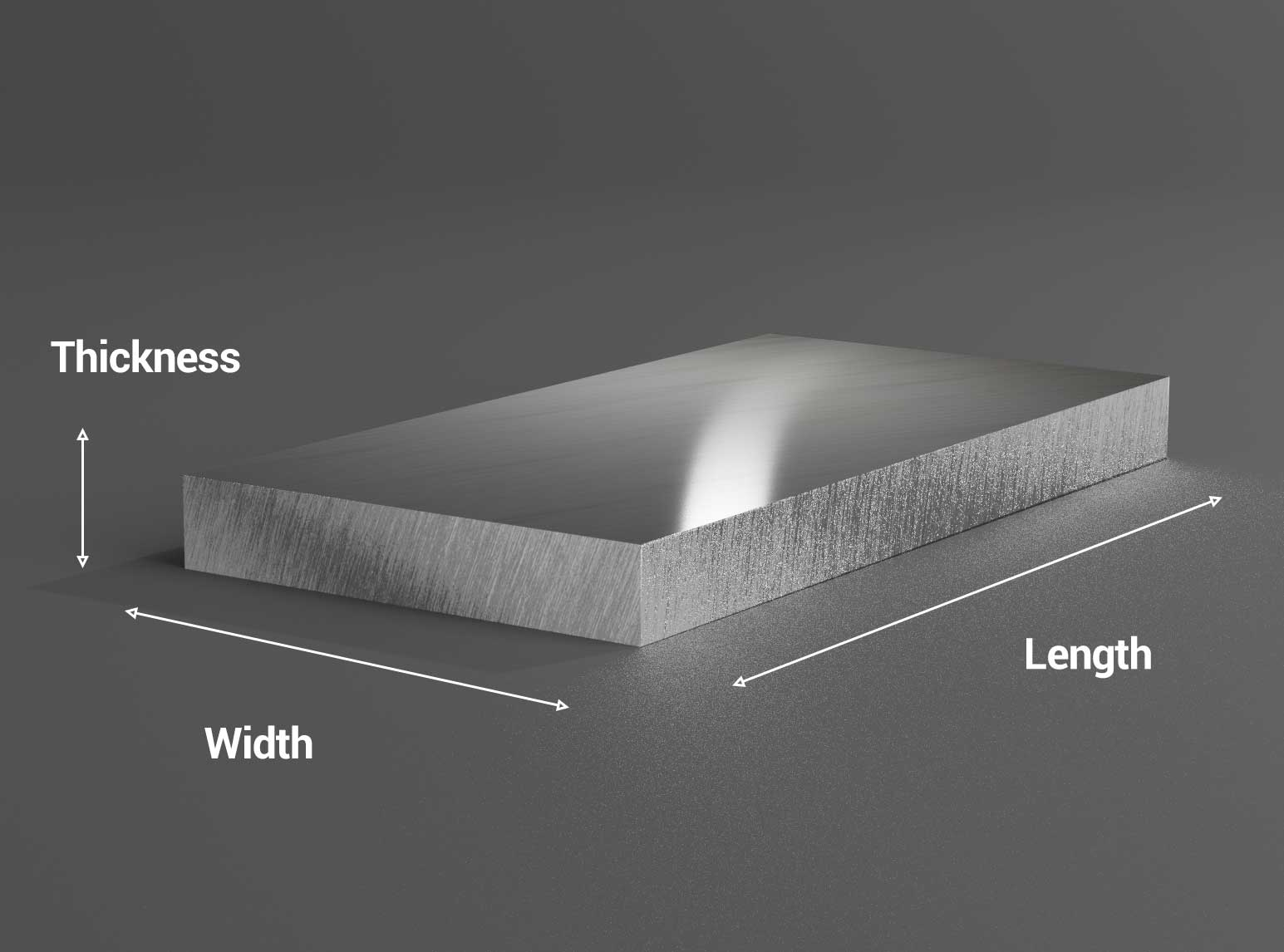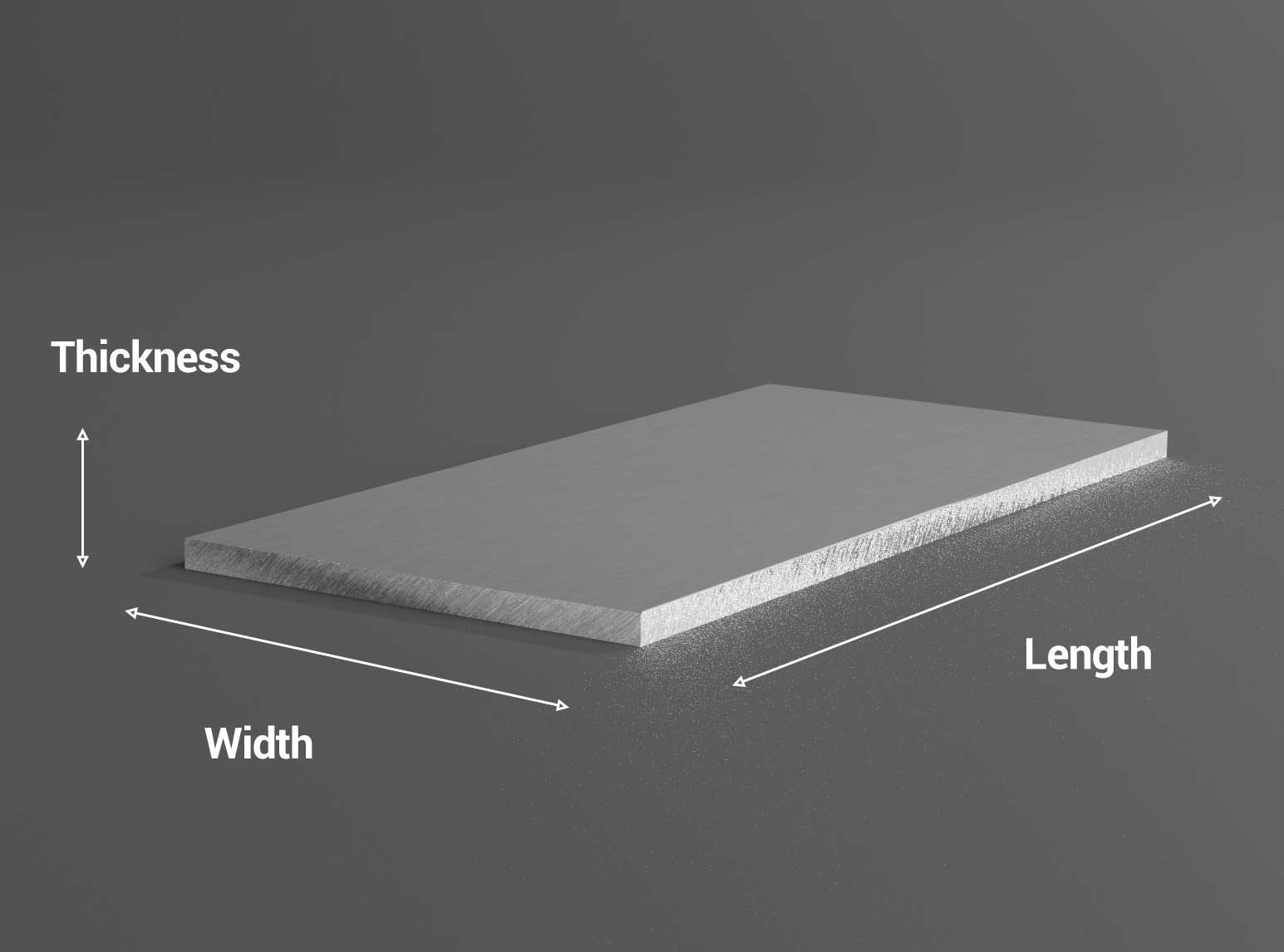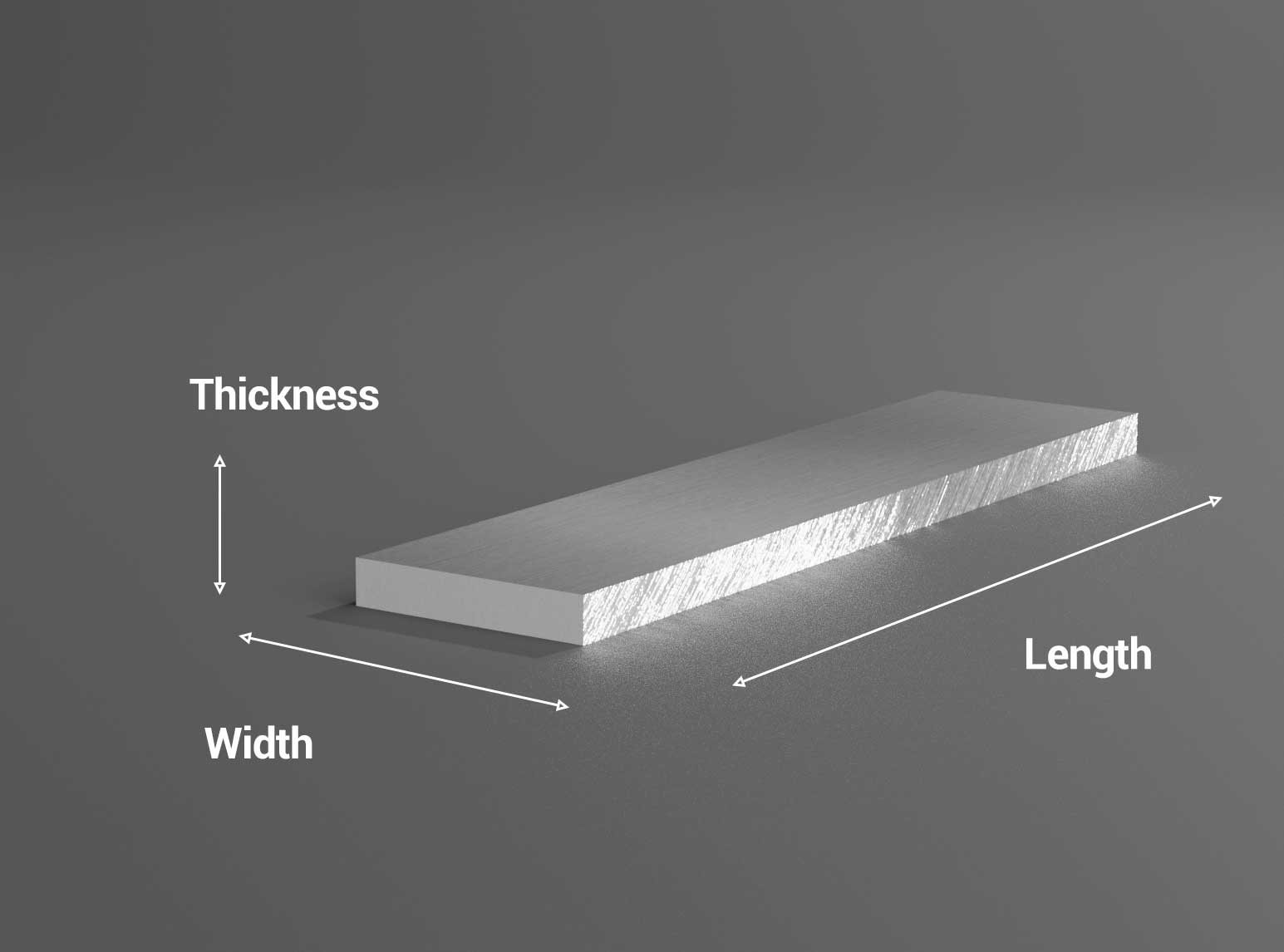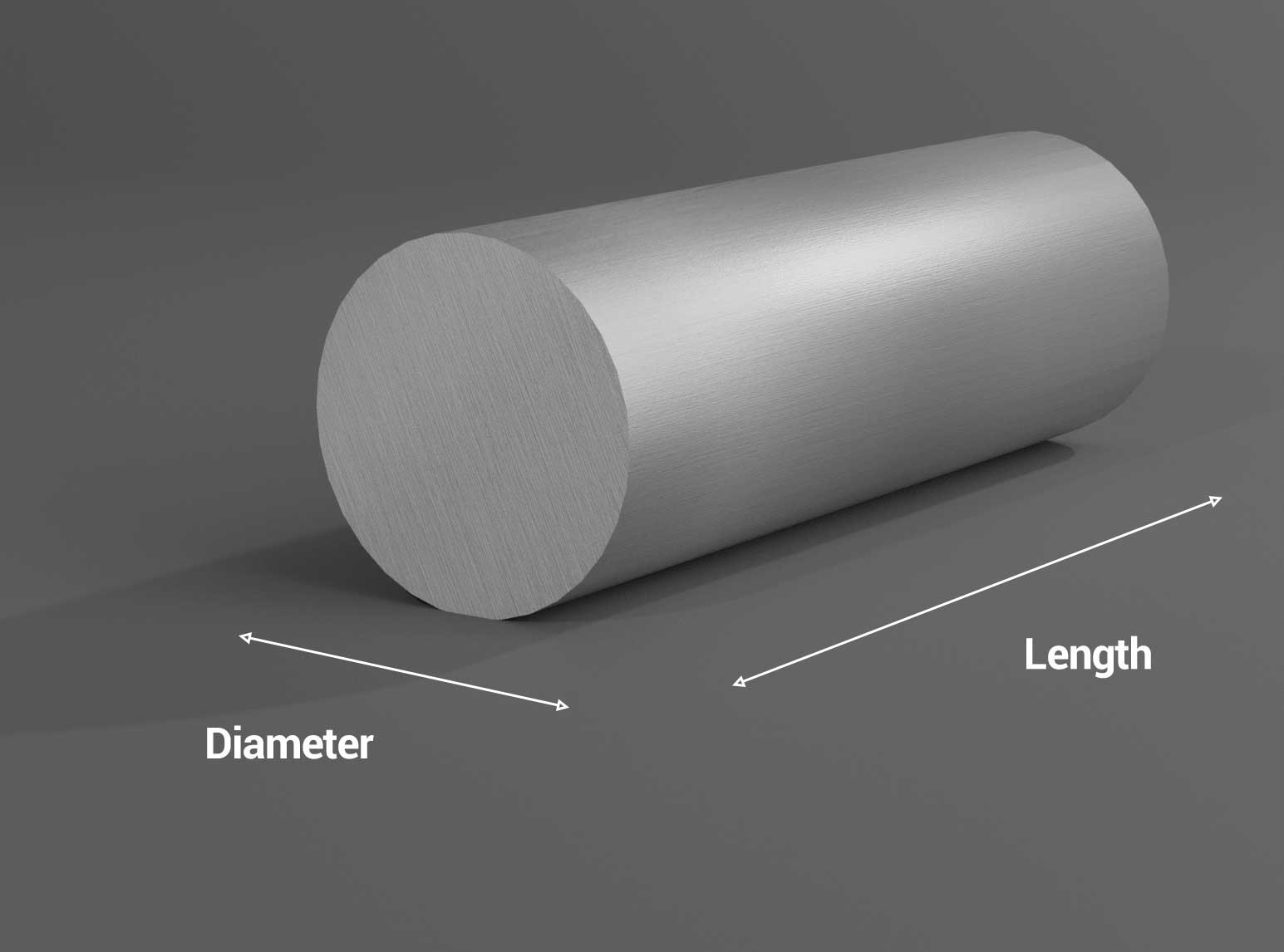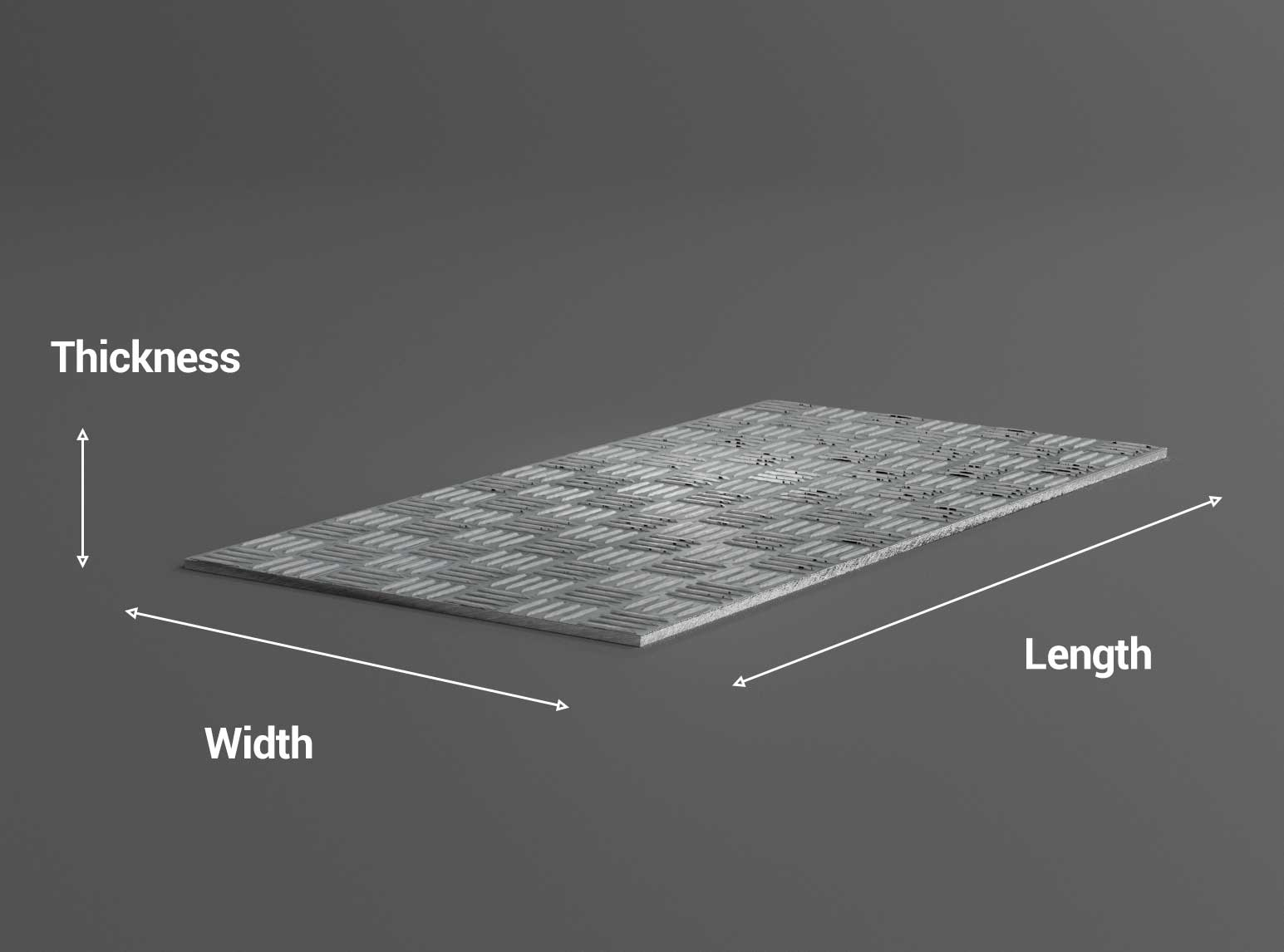Aluminium Weight Calculator
We have used (mm) as the unit of measurement in all of our calculations. In addition to the dimensions, the specific weights of the different alloys have also been factored in to the calculations.
Aluminium Weight Calculation Formula
Our weight calculation formula factors in the thickness, width and height among other dimensions when necessary. It calculates the weight based on the specific alloys properties.... Instead of calculating the lengthy and complicated weight calculation formula yourself, you can gain time by using our aluminium weight calculator.

Sign up for our email newsletter